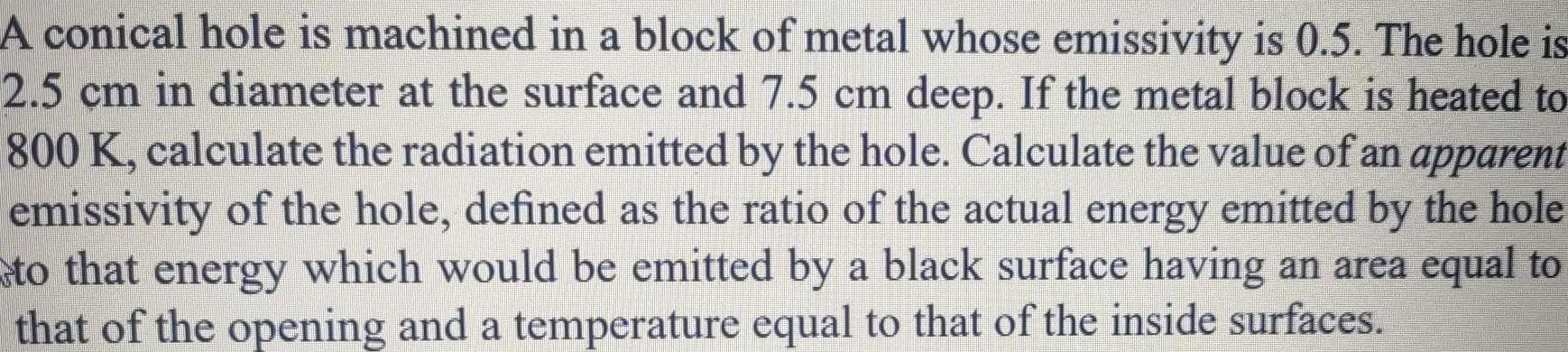Question:

# A conical hole is machined in a block of metal whose

Last updated: 6/8/2023A conical hole is machined in a block of metal whose emissivity is 0 5 The hole is 2 5 cm in diameter at the surface and 7 5 cm deep If the metal block is heated to 800 K calculate the radiation emitted by the hole Calculate the value of an apparent emissivity of the hole defined as the ratio of the actual energy emitted by the hole to that energy which would be emitted by a black surface having an area equal to that of the opening and a temperature equal to that of the inside surfaces